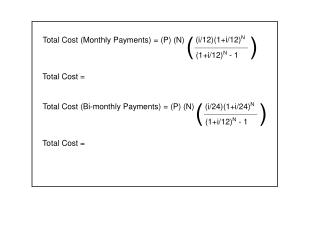DownloadDownload PresentationTotal Cost (Monthly Payments) = (P) (N)

Total Cost (Monthly Payments) = (P) (N)

Download PresentationTotal Cost (Monthly Payments) = (P) (N)

- - - - - - - - - - - - - - - - - - - - - - - - - - - E N D - - - - - - - - - - - - - - - - - - - - - - - - - - -
Presentation Transcript

1. ( ) (i/12)(1+i/12)N Total Cost (Monthly Payments) = (P) (N) (1+i/12)N - 1 Total Cost = ( ) (i/24)(1+i/24)N Total Cost (Bi-monthly Payments) = (P) (N) (1+i/12)N - 1 Total Cost =